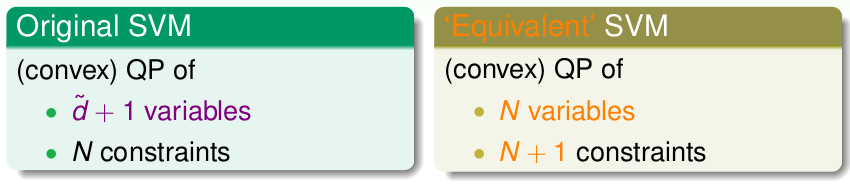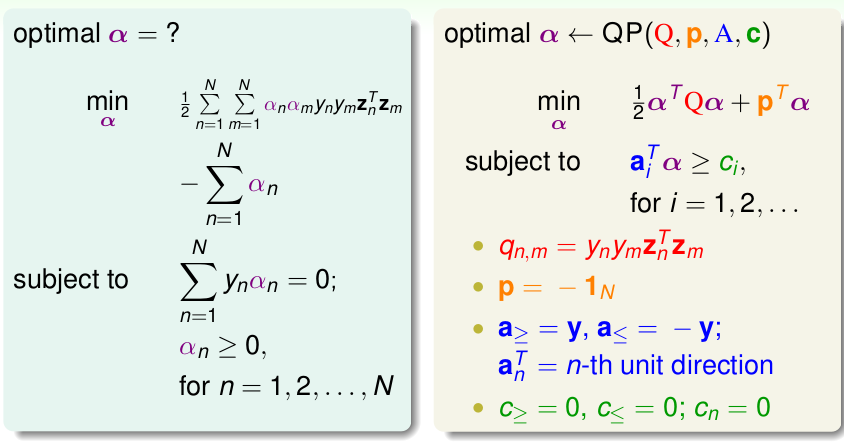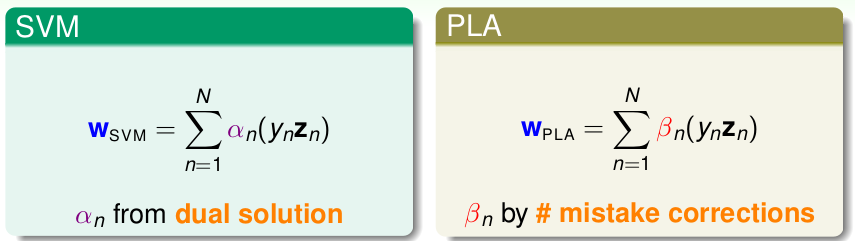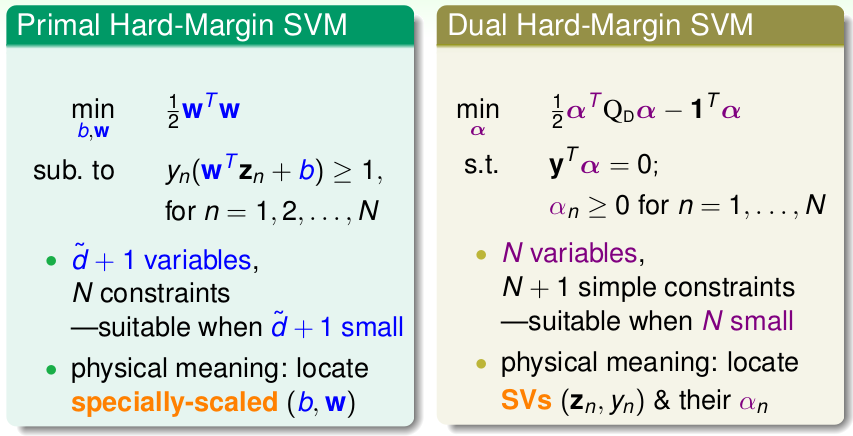# 机器学习技法笔记(3)-对偶SVM

## 对偶的引入### 拉格朗日函数

\begin{align} & \min \limits_{b, \textbf{w}} \quad \frac{1}{2}\textbf{w}^T\textbf{w} \\& s.t. \quad y_n(\textbf{w}^T\textbf{x}+b) \geq 1 \end{align}

$\mathcal{L}(b, \textbf{w}, \mathbf{\alpha}) = \frac{1}{2}\textbf{w}^T \textbf{w} + \sum\limits_{n=1}^{N}\alpha_n(1-y_n(\mathbf{w}^T\mathbf{x}+b)), \quad \alpha_n \geq 0$

$\mathbf{SVM} \equiv \min \limits_{b, \mathbf{w}} (\max \limits_{\alpha_n \geq 0} \mathcal{L}(b, \mathbf{w},\alpha))$

• $\mathbf{w},b$不满足约束条件$y_n(\mathbf{w}^Tx+b) \geq 1$，即: $1-y_n(\mathbf{w}^T\textbf{x}+b) > 0$，再结合$\alpha_n \geq 0$,很显然, $\max(\mathcal{L}) \to \infty$
• $\mathbf{w},b$满足$1-y_n(\mathbf{w}^T\textbf{x}+b) \leq 0$，那么在求$\max$的时候，显然需要$\alpha_n(1-y_n(\mathbf{\mathbf{w}^T\mathbf{x}+b})) = 0$,此时$\max(\mathcal{L}) = \frac{1}{2}\textbf{w}^T \textbf{w}$

### 对偶问题转化

$\min \limits_{b, \mathbf{w}} (\max \limits_{\alpha_n \geq 0} \mathcal{L}(b, \mathbf{w},\alpha)) \geq \min\limits_{b, \mathbf{w}} \mathcal{L}(b, \mathbf{w},\alpha^{'})$

$\min \limits_{b, \mathbf{w}} (\max \limits_{\alpha_n \geq 0} \mathcal{L}(b, \mathbf{w},\alpha)) \geq \max\limits _{all \ \alpha_n \geq 0} (\min\limits_{b, \mathbf{w}} \mathcal{L}(b, \mathbf{w},\alpha^{'}))$

• 原始问题为是凸优化问题 (convex primal)
• 原始问题线性可分的(feasible primal)
• 约束条件是线性的(Linear constraints)

\begin{align*}\max\limits_{all \ \alpha_n \geq 0}{\left ( \min\limits_{b,\mathbf{w}}{ \underbrace{\frac{1}{2}\mathbf{w}^T\mathbf{w}+\sum\limits_{n=1}^{N}\alpha_n(1-y_n(\mathbf{w}^T\mathbf{z}_n+b))}_{\mathcal{L}(b, \mathcal{w}, \alpha)}}\right)}\end{align*}

## 求解对偶问题

### 最小化

\begin{align*} \frac{\partial\mathcal{L}(b, \mathbf{w}, \alpha)}{\partial \ b} = \sum\limits_{n=1}^{N}\alpha_ny_n = 0\end{align*}

$\min\mathcal{L}(b, \mathbf{w},\alpha) = \min \frac{1}{2}\mathbf{w}^T\mathbf{w} + \sum\alpha_n(1-y_n\mathbf{w}^T\mathbf{z}^n) - {b*\sum\alpha_ny_n}$

\begin{align*} \frac{\partial\mathcal{L}(b, \mathbf{w}, \alpha)}{\partial \ \mathbf{w}} = \mathbf{w} - \sum\limits_{n=1}^{N}\alpha_ny_n\mathbf{z}_n = \mathbf{0}\end{align*}

\begin{align} \min\mathcal{L}(b, \mathbf{w},\alpha) & \Leftrightarrow \min \frac{1}{2}\mathbf{w}^T\mathbf{w} + \sum\alpha_n(1-y_n\mathbf{w}^T\mathbf{z}^n) \\ &\Leftrightarrow \min \frac{1}{2}\mathbf{w}^T\mathbf{w} - \sum \alpha_ny_n\mathbf{z}^n\mathbf{w}^T+ \sum\alpha_n \\ & \Leftrightarrow {\min} -\frac{1}{2}\mathbf{w}^T\mathbf{w} + \sum\limits_{n=1}^{N}\alpha_n \\ & \Leftrightarrow -\frac{1}{2} \Arrowvert \sum\limits_{n=1}^{N}\alpha_ny_n\mathbf{z}_n\Arrowvert + \sum\limits_{n=1}^{N}\alpha_{n}\end{align}

\begin{align*}\max\limits_{\alpha_n \geq 0, \ \sum\alpha_ny_n =0, \ \mathbf{w}=\sum\alpha_ny_n\mathbf{z}^n}\left( -\frac{1}{2}\Arrowvert\sum\limits_{n=1}^{N}\alpha_ny_n\mathbf{z}_n\Arrowvert^2+ \sum\limits_{n=1}^{N}\alpha_{n}\right)\end{align*}

### 最大化

\begin{align} \min\limits_{\alpha} & \quad \frac{1}{2}\sum\limits_{n=1}^{N}\sum\limits_{m=1}^{N}{\alpha_n\alpha_m} y_ny_m\mathbf{z}_n\mathbf{z}_m {-}\sum\limits_{n=1}^{N}\alpha_n \\ s.t. & \quad \alpha _n \geq 0, \ \sum\limits_{n=1}^{N}\alpha_ny_n =0 \end{align}• $q_{m,n}$表示矩阵$Q$的第$m$行第$n$列的元素
• 对于$\sum y_n\alpha_n =0$，改为两个不等式条件: $\sum y_n \alpha_n \leq 0,\ \sum y_n\alpha_n \geq 0$,因此一共有$N+2$个约束条件。

### 模型求解

• 对于原始问题有: $y_n(\mathbf{w}^T\mathbf{z}_n+b) \geq 1$
• 对于对偶问题有: $\alpha_n \geq 0$
• 对于对偶问题里层的$\min$优化求解中，有: $\sum \alpha_ny_n =0; \ \mathbf{w}=\sum\alpha_ny_n\mathbf{z}_n$
• 对于原始问题里层的$\max$问题中：上面得到结论有: $\alpha_n(1-y_n(\mathbf{\mathbf{w}^T\mathbf{z}_n+b})) = 0$ ，被称为complementary slackness

$b = y_n - \mathbf{w}^T \mathbf{z}_n, \quad \alpha_n > 0$

1. 根据训练数据，(非线性转换$z_n = \phi(x_n)$， 写出QP问题的$Q,p,a,c$
2. 使用QP Solover 求出最优的$\alpha$
3. 根据条件约束，求出$\mathbf{w},b$
4. 得到模型：$g_{svm} = sign(\mathbf{w}^T\phi(\mathbf{x})+b)$

### 数据表示模型## 后续$Q$矩阵的元素: $q_{n,m} = y_ny_m\mathbf{z}_m\mathbf{z}_n$，实际为$R^{\tilde{d}}$内的内积！ 还是没有摆脱高维。不过对偶问题相比原始问题在非线性数据 的处理上，求解已经更加容易了。 至于如何在求解$q_{n,m}$的时候，彻底不再依赖$\tilde{d}$，那就是下节的内容了，引入带核的SVM。